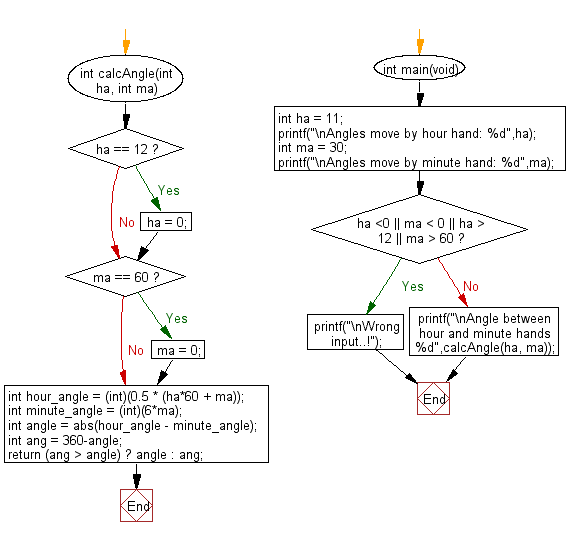﻿ C Program: Find angle between given hour and minute hands - w3resource

# C Exercises: Find angle between given hour and minute hands

## C Programming Mathematics: Exercise-28 with Solution

Write a C program to find the angle between the hour and minute hands.

Example:
Input: int ha = 11
int ma = 30
Output: Angle between hour and minute hands 165

Sample Solution:

C Code:

``````#include <stdio.h>
#include <math.h>

int calcAngle(int ha, int ma)
{
if (ha == 12)
ha = 0;
if (ma == 60)
ma = 0;
int hour_angle = (int)(0.5 * (ha*60 + ma));
int minute_angle = (int)(6*ma);

int angle = abs(hour_angle - minute_angle);
int ang = 360-angle;
return (ang > angle) ? angle : ang;
}

int main(void)

{
int ha = 11;
printf("\nAngles move by hour hand: %d",ha);
int ma = 30;
printf("\nAngles move by minute hand: %d",ma);
if (ha <0 || ma < 0 || ha > 12 || ma > 60)
{
printf("\nWrong input..!");
}
else
{
printf("\nAngle between hour and minute hands %d",calcAngle(ha, ma));
}
}
```
```

Sample Output:

```Angles move by hour hand: 11
Angles move by minute hand: 30
Angle between hour and minute hands 165
```

Flowchart:C Programming Code Editor:

Improve this sample solution and post your code through Disqus.

What is the difficulty level of this exercise?

Test your Programming skills with w3resource's quiz.

﻿